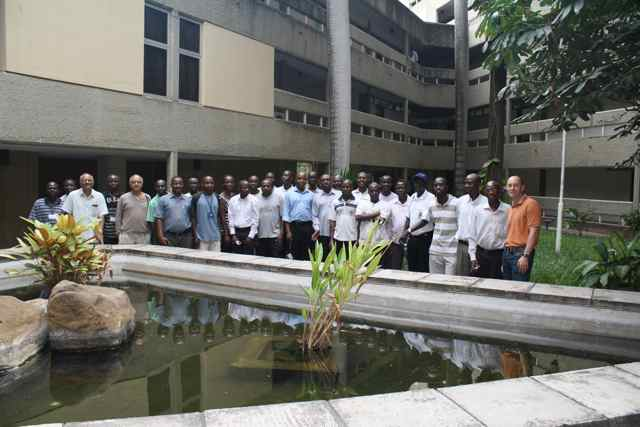Mathematics Summer Schools in Africa Ghana 2003 Cape Coast Kenya 2004 Kenya 2005 Uganda 2006 Tanzania 2007 Linear Algebra

## East African School on Linear Algebra

### December 2-14, 2008 - Mombasa

 Topics and course material The first school Linear dependence, bases, dimension - assuming knowledge of row reduction of linear systems of equations, an assumption that was valid. Application: Existence of interpolation polynomials. Matrix of a linear transformation, kernel and range; how to calculate basis for these using row reduction - free and basic variables. Dimension formula. Membership problem for a subspace. Change of basis. Positive definite matrices, Gram matrices, inner product spaces(some L^2-example), least squares approximations and normal equation. Application: formula for least squares approximation of a line to a point set. Gram-Schmidt, using this to give alternative solution of least squares decomposition. QR-decomposition(but no application) Eigenvalues and eigenvectors, every complex linear map has an eigenvalue. Generalized eigenvalue decomposition. Upper triangular block decomposition (not quite Jordan canonical form, in the process dealing with nilpotent maps, associated filtration(s) and adapted bases. Real spectral theorem(following Axler) Singular value decomposition and pseudoinverse, connection with least squares solutions to linear systems of equations.(following Olver) The second school norm of a linear operator on C^n; and different expressions for it (as max ||A(x)||, as max |x*Ay|, as the maximum of singular values; etc. examples of some other norms; their merits and demerits; eg the operator norm is very difficult to evaluate even in low dimensions, the Hilbert-Schmidt norm is easy but does not always make sense in infinite dimensions. special properties of Hermitian, normal, unitary matrices some facility with diagonalisation and its uses Schur's theorem that every matrix is unitarily equivalent to an upper triangular matrix. some idea of "invariance" of various objects under transformations; eg similarity would preserve trace, det, eigenvalues, invertibility, rank but not norm, singular values, hermiticity. counting of dimensions of subspaces and their intersections. (just simple things like if two or more subspaces have sufficiently high dimensions then their intersection is nonzero.) the google page rank algorithm Inequalities for sums of eigenvalues of Hermitian matrices (starting with Weyl, going through various minmax principles, and an allusion to Horn's problem) statements of these as perturbation bounds the problems, solved and unsolved for non-Hermitian matrices continuity of roots of polynomials and of eigenvalues---qualitative and quantitative Instructors The first school Rikard Bogvad Ramadas Trivandrum The second school Rajendra Bhatia Mike Zabrocki Ramadas Trivandrum Additional material Google's PageRank Algorithm Wikipedia article about Google PageRank algorithm M. Bianchini, M. Gori, F. Scarseli, Inside PageRank, ACM Transactions on Internet Technology, Vol. 5, No. 1, February 2005, pp. 92-128. A. N. Langville and C. D. Meyer, Deeper Inside PageRank, Internet Mathematics Vol. I, No. 3, pp. 335-380. K. Bryan and T. Leise, The \$25,000,000,000 Eigenvector: The Linear Algebra Behind Google, SIAM Review, Vol. 48, No. 3. (2006), pp. 569-581. Group pictures from this eventPicture 1 - Medium (204K) Large (4.4MB) Picture 2 - Medium (204K) Large (4.4MB) Picture 3 - Medium (204K) Large (4.4MB)

This event was sponsored by the International Center for Theoretical Physics and the Swedish International Development Cooperation Agency.

 Mathematics Summer Schools in Africa Ghana 2003 Cape Coast Kenya 2004 Kenya 2005 Uganda 2006 Tanzania 2007 Linear Algebra